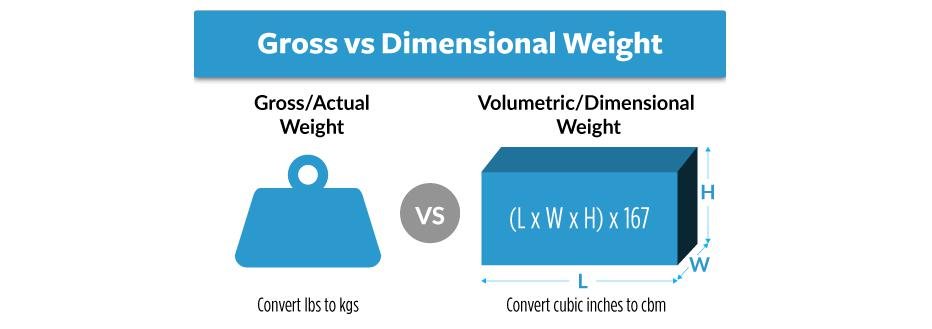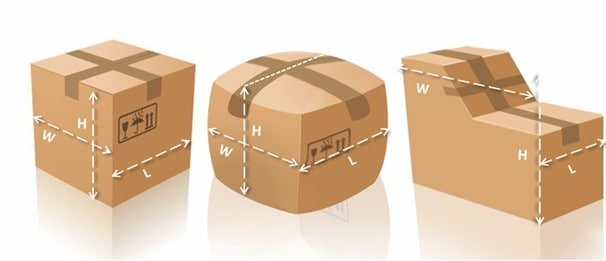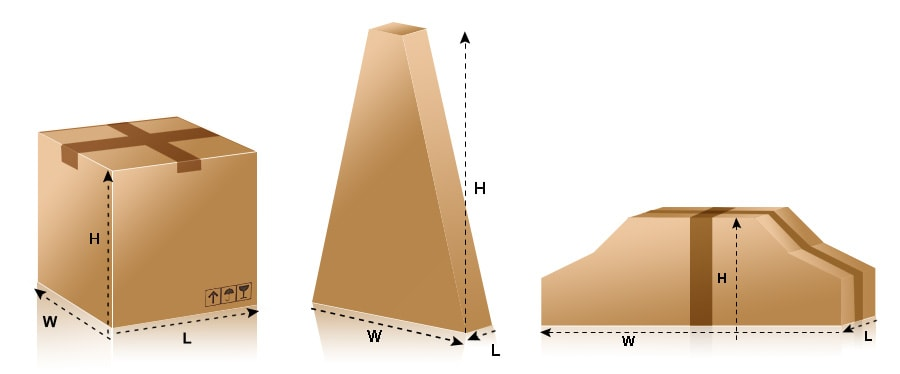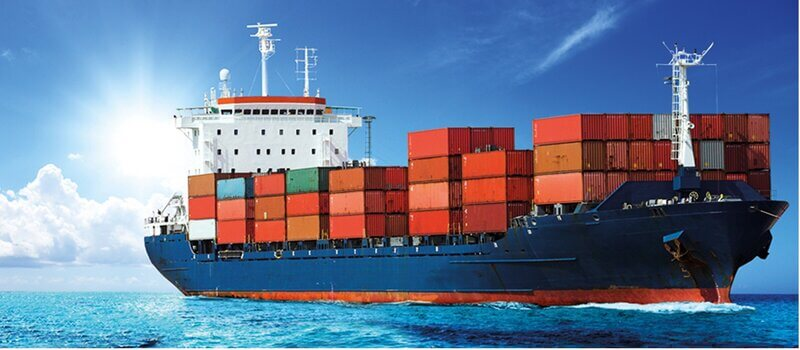# How to Calculate Chargeable Weight for Air & Sea Freight Shipments?

Why freight forwarder companies do not use gross weight or volumetric weight of the cargo but the chargeable weight when calculating freight cost?

You see, some goods are heavy, but take very limited space; whereas others are really light but allocates tons of room in the transportation.

Imagine a shipment of cotton and steel. It is quite obvious that a cotton shipment will occupy more space compared to a steel shipment. However, a steel shipment will be weighing more. Should both be charged using volume Weight?They choose the higher value between the volumetric weight and the actual weight of the cargo. This is fair enough because cotton shipments will occupy more space while steel shipments will weigh more. In both cases, shippers will be choosing the measurement with greater value.

## What is the calculation formula for Chargeable Weight?

For those of you who simply want the formulas without a detailed explanation, here we go:

## Gross Weight VS Volumetric Weight

In international logistics, goods will be divided into light weighted goods and heavy goods, then what is light weighted goods, the difference between heavy goods and heavy goods?  To understand the definition of light goods and heavy goods, we need to know what is the actual gross weight, volume weight, and chargeable weight.

### The Actual Weight:

Actual Weight, which is the weight obtained from weighing (overweight), including Gross Weight (G.W.) and Net Weight (N.W.). The most common is the actual gross weight.

In air cargo transportation, the actual gross weight is often compared to the calculated volumetric weight, whichever is calculated and charged.

### The Volume Weight

Volume Weight, also named as Volumetric Weight or Dimensions Weight, which is the weight calculated from the volume of the cargo according to a certain conversion factor or calculation formula.

In international freight transportation, the freight is calculated on the basis of which value is large between the actual weight and the volumetric weight of the goods.

Normally, light loads are charged by dimensional weight, while heavier loads are charged by actual weight.# Chargeable Weight

Chargeable Weight, referred to as C.W., which is used to calculate the weight of freight or other miscellaneous charges.

The chargeable weight is either the actual gross weight or the volume weight, the chargeable weight = the actual weight VS the volume weight, and the larger one is the weight for calculating the shipping cost.

## How does air freight company calculate the Chargeable Weight?

In air freight transportation, the calculation factor for calculating the volumetric weight is generally 1:167, that is, one cubic meter is equal to about 167 kilograms.

For example, the actual gross weight of an air cargo shipment is 95 kg, and the volume is 1.2 cubic meters. Calculated according to the coefficient of air transportation 1:167, the volume weight of this cargo is 1.2*167=200.4 kg, which is greater than the actual gross weight of 95 kg, so this cargo (also called soft, light goods, English called Light Weight Cargo or Light Cargo/Goods or Low Density Cargo or Measurement Cargo), air transport will be charged by volume, not actual gross weight.

Another example: the actual gross weight of a shipment air cargo is 560 kg, the volume is 1.5 CBM, calculated according to the coefficient of air transport 1:167, the volume weight of the ticket is 1.5 * 167 = 250.5 kg, less than the actual gross weight of 560 kg, so this cargo is named as heavy goods (English called Heavy Cargo/Goods or High Density Cargo), the airline companies will charge according to the actual gross weight, not the volume weight.

In short, according to a certain conversion coefficient, calculate the volume weight, and then compare the volume weight with the actual weight, whichever is larger will be charged according to that.

### Regular items:

Length (cm) x width (cm) x height (cm) ÷ 6000 = volumetric weight (KG), ie 1 CBM ≈ 166.6666 KG.

### Irregular items:Irregularly Shaped Air freight Shipment

The longest (cm) x widest (cm) x highest (cm) ÷ 6000 = volumetric weight (KG), ie 1 CBM ≈ 166.666667 KG.

This is an internationally accepted algorithm.

In short, 1 cubic meter of weight greater than 166.67 kilograms is called heavy cargo, and less than 166.67 kilograms is called foam cargo in China.

Heavy goods are charged according to the actual gross weight, and the foamed goods are charged according to the volumetric weight.

### Air Freight Chargeable Weight Calcuation:

The gross weight of an air shipment can be higher in value compared to its volumetric weight. This makes it the chosen chargeable weight for the cargo as shown in the below example.

Shipment measurement:

• Length (l) = 80cm
• Width (w) = 20cm
• Height (h) = 45cm
• Gross Weight = 5Kgs

Step 1; Calculate the volume weight of the air freight shipment

Volume weight= length X width X height
= 80cm x 20cmx 45cm
= 11.4Kg

Step 2; Compare the gross weight with the volumetric weight

volumetric weight vs gross weight = 11.4 kgs vs 9.5kgs

Chargeable weight is the greater value which is 11.4 kgs.

## How does Express Freight calculate Chargeable Weight?

Express freight calculation methods:

There are three ways to measure the volume in practice:

### 1 Standard package measurement method:

The package is with a standard carton size,

The volume is calculated according to the length, height and width of the carton.

For example, DHL channel, package length 15cm, width 30cm, height 20cm,

Then the volume weight = (15 × 30 × 20) / 5000 = 1.8KG,

The actual weight is 1.2KG,

Then the parcel demensional weight calculated is 1.8KG.

The final DHL chargeable weight is 2KG.### 2 Surface package measurement method:

If the package is squeezed by the goods, the plane of the box expands and deforms,

Then calculate according to the longest side of the package.

For example, DHL channel, the actual length of the carton is 15cm, 30cm, and 20cm;

Due to the extrusion of the goods, the length is 18cm, 33cm, and 22cm after sealing.

Then the volume weight = (18 × 33 × 22) / 5000 = 2.7 KG,

The actual weight is 2KG,

Then the package calculates the shipping cost by the volume of 2.7KG.

The final billing weight is 3KG.

### 3 Irregular package measurement method:

Due to the actual size of the goods, it needs to be packaged into irregular packages.

Then the package is calculated according to the longest side and the volume is heavy.

Example: such as the UPS channel,

Irregular parcels have a maximum length of 15cm, a maximum length of 40cm, and a maximum height of 25cm.

Then the volume weight= (15 × 40 × 25) / 5000 = 3KG,

The actual weight is 2KG,

Finally, the package calculates the shipping cost by the volume weight of 3KG.

However, what customers often overlook is the design of the box itself. The size indicated on the box is the inner diameter of the box, it does not contain the thickness of the paper, but actual shipment is measured by the outer dimensions of the box.

### Notices:

1. CBM is the abbreviation of Cubic Meter, which means cubic meter.

2. Only the express (DHL, FedEx, TNT, UPS, Aramax) company uses this algorithm length (cm) × width (cm) × height (cm) ÷ 5000 to calculate the volume weight.

3. In order to make full use of the space and load capacity of the airplane,the heavy cargo and the light cargo will generally be reasonably matched. The air cargo loading is a technical activity – well matched, can make full use of the limited space of the aircraft, and if do well, it can greatly increase the extra profit. If there are too many heavy goods, it will waste space (it will be overweight without filling the space). Too much cargo will waste the load (filled with the maximum weight).

## Sea Freight Chargeable Weight CalculationThe division of heavy goods and light goods by sea freight is much simpler than that of air transportation. China’s sea freight consolidation business basically divides heavy goods and light goods according to the standard of 1 cubic meter equal to 1 ton(1CBM=1000KG). In the LCL ocean freight, heavy goods are rare, basically light goods, and shipping LCL is calculated according to the volume of freight, compared with air transport according to the weight of the freight is fundamentally different, so it is much simpler. Many people have done a lot of shipping, but have never heard of light goods and heavy goods, because they are basically not used.

According to the stowage of the ship, any cargo with a cargo stowage factor less than the ship’s capacity factor is called Heavy Weight Cargo; any cargo with a cargo stowage factor greater than the ship’s tank capacity is called Measurement Cargo/Light Goods.

According to the calculation of freight aspectand international shipping business practices, any cargo with a stowage factor of less than 1.1328 m3/ton or 40 cubic feet/ton is called heavy cargo; where the cargo stowage factor is greater than 1.1328 m3/ton or 40 Cubic feet per ton of cargo, called light goods / foamgoods.

The concept of heavy goods and light goods is closely related to stowage, transportation, storage and billing. The carrier or freight forwarder company distinguishes heavy goods, light goods/foam goods according to certain standards.

Do you need any assistance moving your cargo either via air or sea? We are happy to help you, so contact us now and send a message for a quote on doing your air or sea freight from China.